# Huffman Coding

Huffman Coding is a technique of compressing data to reduce its size without losing any of the details. It was first developed by David Huffman.

Huffman Coding is generally useful to compress the data in which there are frequently occurring characters.

## How Huffman Coding works?

Suppose the string below is to be sent over a network.

Each character occupies 8 bits. There are a total of 15 characters in the above string. Thus, a total of `8 * 15 = 120` bits are required to send this string.

Using the Huffman Coding technique, we can compress the string to a smaller size.

Huffman coding first creates a tree using the frequencies of the character and then generates code for each character.

Once the data is encoded, it has to be decoded. Decoding is done using the same tree.

Huffman Coding prevents any ambiguity in the decoding process using the concept of prefix code ie. a code associated with a character should not be present in the prefix of any other code. The tree created above helps in maintaining the property.

Huffman coding is done with the help of the following steps.

1. Calculate the frequency of each character in the string.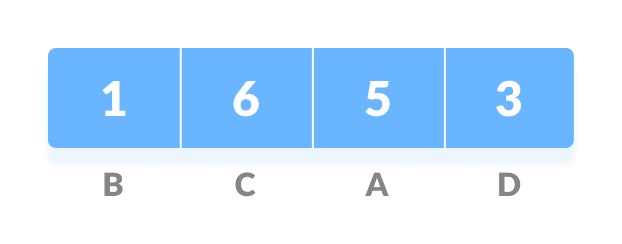Frequency of string
2. Sort the characters in increasing order of the frequency. These are stored in a priority queue Q.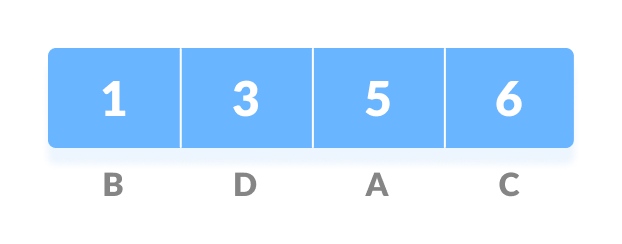Characters sorted according to the frequency
3. Make each unique character as a leaf node.
4. Create an empty node z. Assign the minimum frequency to the left child of z and assign the second minimum frequency to the right child of z. Set the value of the z as the sum of the above two minimum frequencies.Getting the sum of the least numbers
5. Remove these two minimum frequencies from Q and add the sum into the list of frequencies (* denote the internal nodes in the figure above).
6. Insert node z into the tree.
7. Repeat steps 3 to 5 for all the characters.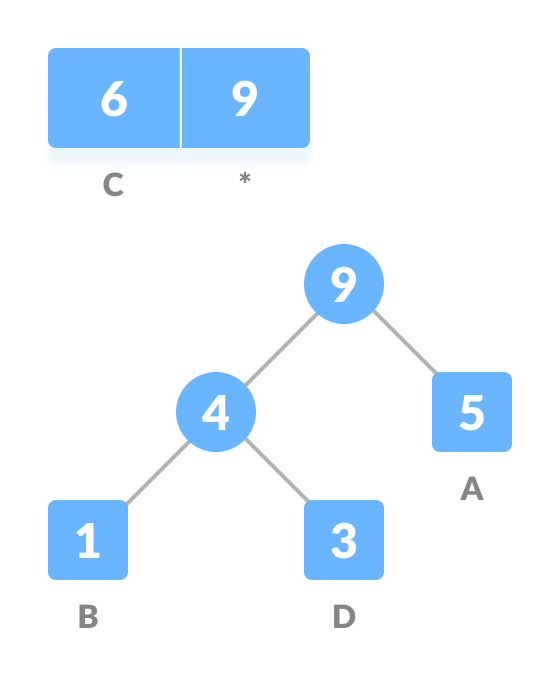Repeat steps 3 to 5 for all the characters.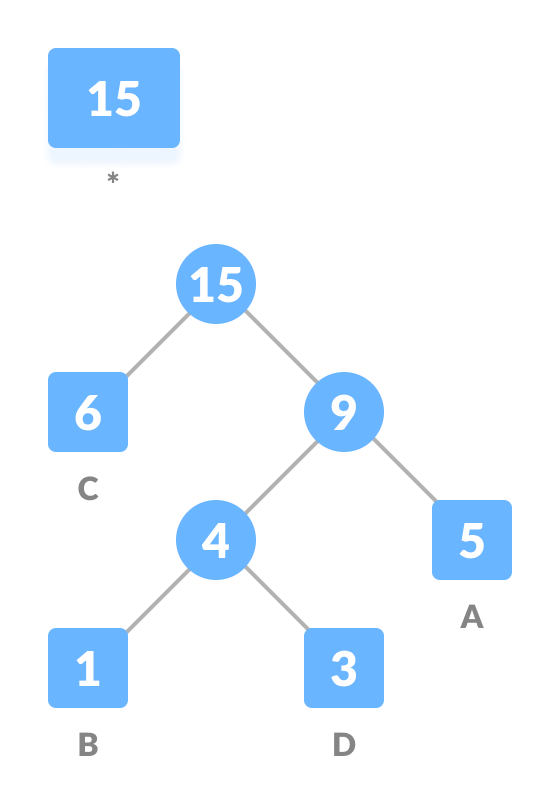Repeat steps 3 to 5 for all the characters.
8. For each non-leaf node, assign 0 to the left edge and 1 to the right edge.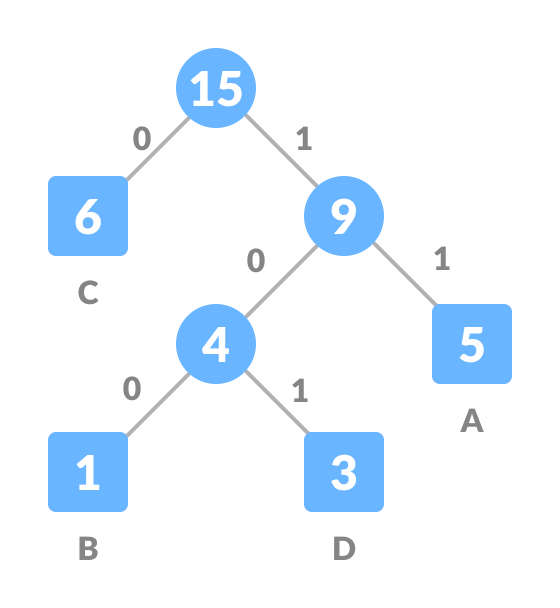Assign 0 to the left edge and 1 to the right edge

For sending the above string over a network, we have to send the tree as well as the above compressed-code. The total size is given by the table below.

Character Frequency Code Size
A 5 11 5*2 = 10
B 1 100 1*3 = 3
C 6 0 6*1 = 6
D 3 101 3*3 = 9
4 * 8 = 32 bits 15 bits   28 bits

Without encoding, the total size of the string was 120 bits. After encoding the size is reduced to `32 + 15 + 28 = 75`.

## Decoding the code

For decoding the code, we can take the code and traverse through the tree to find the character.

Let 101 is to be decoded, we can traverse from the root as in the figure below.

## Huffman Coding Algorithm

```create a priority queue Q consisting of each unique character.
sort then in ascending order of their frequencies.
for all the unique characters:
create a newNode
extract minimum value from Q and assign it to leftChild of newNode
extract minimum value from Q and assign it to rightChild of newNode
calculate the sum of these two minimum values and assign it to the value of newNode
insert this newNode into the tree
return rootNode```

## Python, Java and C/C++ Examples

``````# Huffman Coding in python

# Creating tree nodes
class NodeTree(object):

def __init__(self, left=None, right=None):
self.left = left
self.right = right

def children(self):
return (self.left, self.right)

def nodes(self):
return (self.left, self.right)

def __str__(self):
return '%s_%s' % (self.left, self.right)

# Main function implementing huffman coding
def huffman_code_tree(node, left=True, binString=''):
if type(node) is str:
return {node: binString}
(l, r) = node.children()
d = dict()
d.update(huffman_code_tree(l, True, binString + '0'))
d.update(huffman_code_tree(r, False, binString + '1'))
return d

# Calculating frequency
freq = {}
for c in string:
if c in freq:
freq[c] += 1
else:
freq[c] = 1

freq = sorted(freq.items(), key=lambda x: x, reverse=True)

nodes = freq

while len(nodes) > 1:
(key1, c1) = nodes[-1]
(key2, c2) = nodes[-2]
nodes = nodes[:-2]
node = NodeTree(key1, key2)
nodes.append((node, c1 + c2))

nodes = sorted(nodes, key=lambda x: x, reverse=True)

huffmanCode = huffman_code_tree(nodes)

print(' Char | Huffman code ')
print('----------------------')
for (char, frequency) in freq:
print(' %-4r |%12s' % (char, huffmanCode[char]))``````
``````// Huffman Coding in Java

import java.util.PriorityQueue;
import java.util.Comparator;

class HuffmanNode {
int item;
char c;
HuffmanNode left;
HuffmanNode right;
}

// For comparing the nodes
class ImplementComparator implements Comparator<HuffmanNode> {
public int compare(HuffmanNode x, HuffmanNode y) {
return x.item - y.item;
}
}

// IMplementing the huffman algorithm
public class Huffman {
public static void printCode(HuffmanNode root, String s) {
if (root.left == null && root.right == null && Character.isLetter(root.c)) {

System.out.println(root.c + "   |  " + s);

return;
}
printCode(root.left, s + "0");
printCode(root.right, s + "1");
}

public static void main(String[] args) {

int n = 4;
char[] charArray = { 'A', 'B', 'C', 'D' };
int[] charfreq = { 5, 1, 6, 3 };

PriorityQueue<HuffmanNode> q = new PriorityQueue<HuffmanNode>(n, new ImplementComparator());

for (int i = 0; i < n; i++) {
HuffmanNode hn = new HuffmanNode();

hn.c = charArray[i];
hn.item = charfreq[i];

hn.left = null;
hn.right = null;

}

HuffmanNode root = null;

while (q.size() > 1) {

HuffmanNode x = q.peek();
q.poll();

HuffmanNode y = q.peek();
q.poll();

HuffmanNode f = new HuffmanNode();

f.item = x.item + y.item;
f.c = '-';
f.left = x;
f.right = y;
root = f;

}
System.out.println(" Char | Huffman code ");
System.out.println("--------------------");
printCode(root, "");
}
}``````
``````// Huffman Coding in C

#include <stdio.h>
#include <stdlib.h>

#define MAX_TREE_HT 50

struct MinHNode {
char item;
unsigned freq;
struct MinHNode *left, *right;
};

struct MinHeap {
unsigned size;
unsigned capacity;
struct MinHNode **array;
};

// Create nodes
struct MinHNode *newNode(char item, unsigned freq) {
struct MinHNode *temp = (struct MinHNode *)malloc(sizeof(struct MinHNode));

temp->left = temp->right = NULL;
temp->item = item;
temp->freq = freq;

return temp;
}

// Create min heap
struct MinHeap *createMinH(unsigned capacity) {
struct MinHeap *minHeap = (struct MinHeap *)malloc(sizeof(struct MinHeap));

minHeap->size = 0;

minHeap->capacity = capacity;

minHeap->array = (struct MinHNode **)malloc(minHeap->capacity * sizeof(struct MinHNode *));
return minHeap;
}

// Function to swap
void swapMinHNode(struct MinHNode **a, struct MinHNode **b) {
struct MinHNode *t = *a;
*a = *b;
*b = t;
}

// Heapify
void minHeapify(struct MinHeap *minHeap, int idx) {
int smallest = idx;
int left = 2 * idx + 1;
int right = 2 * idx + 2;

if (left < minHeap->size && minHeap->array[left]->freq < minHeap->array[smallest]->freq)
smallest = left;

if (right < minHeap->size && minHeap->array[right]->freq < minHeap->array[smallest]->freq)
smallest = right;

if (smallest != idx) {
swapMinHNode(&minHeap->array[smallest], &minHeap->array[idx]);
minHeapify(minHeap, smallest);
}
}

// Check if size if 1
int checkSizeOne(struct MinHeap *minHeap) {
return (minHeap->size == 1);
}

// Extract min
struct MinHNode *extractMin(struct MinHeap *minHeap) {
struct MinHNode *temp = minHeap->array;
minHeap->array = minHeap->array[minHeap->size - 1];

--minHeap->size;
minHeapify(minHeap, 0);

return temp;
}

// Insertion function
void insertMinHeap(struct MinHeap *minHeap, struct MinHNode *minHeapNode) {
++minHeap->size;
int i = minHeap->size - 1;

while (i && minHeapNode->freq < minHeap->array[(i - 1) / 2]->freq) {
minHeap->array[i] = minHeap->array[(i - 1) / 2];
i = (i - 1) / 2;
}
minHeap->array[i] = minHeapNode;
}

void buildMinHeap(struct MinHeap *minHeap) {
int n = minHeap->size - 1;
int i;

for (i = (n - 1) / 2; i >= 0; --i)
minHeapify(minHeap, i);
}

int isLeaf(struct MinHNode *root) {
return !(root->left) && !(root->right);
}

struct MinHeap *createAndBuildMinHeap(char item[], int freq[], int size) {
struct MinHeap *minHeap = createMinH(size);

for (int i = 0; i < size; ++i)
minHeap->array[i] = newNode(item[i], freq[i]);

minHeap->size = size;
buildMinHeap(minHeap);

return minHeap;
}

struct MinHNode *buildHuffmanTree(char item[], int freq[], int size) {
struct MinHNode *left, *right, *top;
struct MinHeap *minHeap = createAndBuildMinHeap(item, freq, size);

while (!checkSizeOne(minHeap)) {
left = extractMin(minHeap);
right = extractMin(minHeap);

top = newNode('\$', left->freq + right->freq);

top->left = left;
top->right = right;

insertMinHeap(minHeap, top);
}
return extractMin(minHeap);
}

void printHCodes(struct MinHNode *root, int arr[], int top) {
if (root->left) {
arr[top] = 0;
printHCodes(root->left, arr, top + 1);
}
if (root->right) {
arr[top] = 1;
printHCodes(root->right, arr, top + 1);
}
if (isLeaf(root)) {
printf("  %c   | ", root->item);
printArray(arr, top);
}
}

// Wrapper function
void HuffmanCodes(char item[], int freq[], int size) {
struct MinHNode *root = buildHuffmanTree(item, freq, size);

int arr[MAX_TREE_HT], top = 0;

printHCodes(root, arr, top);
}

// Print the array
void printArray(int arr[], int n) {
int i;
for (i = 0; i < n; ++i)
printf("%d", arr[i]);

printf("\n");
}

int main() {
char arr[] = {'A', 'B', 'C', 'D'};
int freq[] = {5, 1, 6, 3};

int size = sizeof(arr) / sizeof(arr);

printf(" Char | Huffman code ");
printf("\n--------------------\n");

HuffmanCodes(arr, freq, size);
}``````
``````// Huffman Coding in C++

#include <iostream>
using namespace std;

#define MAX_TREE_HT 50

struct MinHNode {
unsigned freq;
char item;
struct MinHNode *left, *right;
};

struct MinH {
unsigned size;
unsigned capacity;
struct MinHNode **array;
};

// Creating Huffman tree node
struct MinHNode *newNode(char item, unsigned freq) {
struct MinHNode *temp = (struct MinHNode *)malloc(sizeof(struct MinHNode));

temp->left = temp->right = NULL;
temp->item = item;
temp->freq = freq;

return temp;
}

// Create min heap using given capacity
struct MinH *createMinH(unsigned capacity) {
struct MinH *minHeap = (struct MinH *)malloc(sizeof(struct MinH));
minHeap->size = 0;
minHeap->capacity = capacity;
minHeap->array = (struct MinHNode **)malloc(minHeap->capacity * sizeof(struct MinHNode *));
return minHeap;
}

// Print the array
void printArray(int arr[], int n) {
int i;
for (i = 0; i < n; ++i)
cout << arr[i];

cout << "\n";
}

// Swap function
void swapMinHNode(struct MinHNode **a, struct MinHNode **b) {
struct MinHNode *t = *a;
*a = *b;
*b = t;
}

// Heapify
void minHeapify(struct MinH *minHeap, int idx) {
int smallest = idx;
int left = 2 * idx + 1;
int right = 2 * idx + 2;

if (left < minHeap->size && minHeap->array[left]->freq < minHeap->array[smallest]->freq)
smallest = left;

if (right < minHeap->size && minHeap->array[right]->freq < minHeap->array[smallest]->freq)
smallest = right;

if (smallest != idx) {
swapMinHNode(&minHeap->array[smallest],
&minHeap->array[idx]);
minHeapify(minHeap, smallest);
}
}

// Check if size if 1
int checkSizeOne(struct MinH *minHeap) {
return (minHeap->size == 1);
}

// Extract the min
struct MinHNode *extractMin(struct MinH *minHeap) {
struct MinHNode *temp = minHeap->array;
minHeap->array = minHeap->array[minHeap->size - 1];

--minHeap->size;
minHeapify(minHeap, 0);

return temp;
}

// Insertion
void insertMinHeap(struct MinH *minHeap, struct MinHNode *minHeapNode) {
++minHeap->size;
int i = minHeap->size - 1;

while (i && minHeapNode->freq < minHeap->array[(i - 1) / 2]->freq) {
minHeap->array[i] = minHeap->array[(i - 1) / 2];
i = (i - 1) / 2;
}

minHeap->array[i] = minHeapNode;
}

// BUild min heap
void buildMinHeap(struct MinH *minHeap) {
int n = minHeap->size - 1;
int i;

for (i = (n - 1) / 2; i >= 0; --i)
minHeapify(minHeap, i);
}

int isLeaf(struct MinHNode *root) {
return !(root->left) && !(root->right);
}

struct MinH *createAndBuildMinHeap(char item[], int freq[], int size) {
struct MinH *minHeap = createMinH(size);

for (int i = 0; i < size; ++i)
minHeap->array[i] = newNode(item[i], freq[i]);

minHeap->size = size;
buildMinHeap(minHeap);

return minHeap;
}

struct MinHNode *buildHfTree(char item[], int freq[], int size) {
struct MinHNode *left, *right, *top;
struct MinH *minHeap = createAndBuildMinHeap(item, freq, size);

while (!checkSizeOne(minHeap)) {
left = extractMin(minHeap);
right = extractMin(minHeap);

top = newNode('\$', left->freq + right->freq);

top->left = left;
top->right = right;

insertMinHeap(minHeap, top);
}
return extractMin(minHeap);
}
void printHCodes(struct MinHNode *root, int arr[], int top) {
if (root->left) {
arr[top] = 0;
printHCodes(root->left, arr, top + 1);
}

if (root->right) {
arr[top] = 1;
printHCodes(root->right, arr, top + 1);
}
if (isLeaf(root)) {
cout << root->item << "  | ";
printArray(arr, top);
}
}

// Wrapper function
void HuffmanCodes(char item[], int freq[], int size) {
struct MinHNode *root = buildHfTree(item, freq, size);

int arr[MAX_TREE_HT], top = 0;

printHCodes(root, arr, top);
}

int main() {
char arr[] = {'A', 'B', 'C', 'D'};
int freq[] = {5, 1, 6, 3};

int size = sizeof(arr) / sizeof(arr);

cout << "Char | Huffman code ";
cout << "\n----------------------\n";
HuffmanCodes(arr, freq, size);
}``````

## Huffman Coding Complexity

The time complexity for encoding each unique character based on its frequency is `O(nlog n)`.

Extracting minimum frequency from the priority queue takes place `2*(n-1)` times and its complexity is `O(log n)`. Thus the overall complexity is `O(nlog n)`.

## Huffman Coding Applications

• Huffman coding is used in conventional compression formats like GZIP, BZIP2, PKZIP, etc.
• For text and fax transmissions.